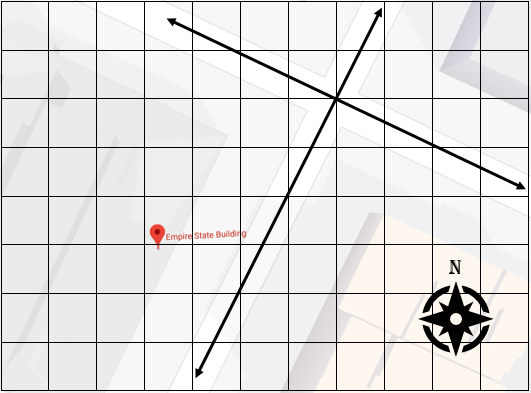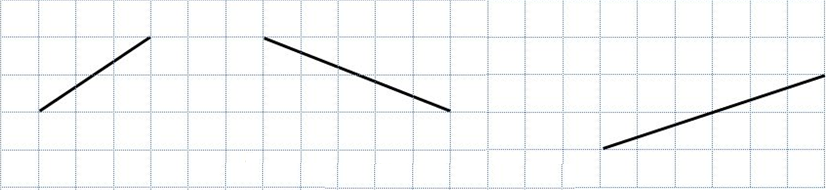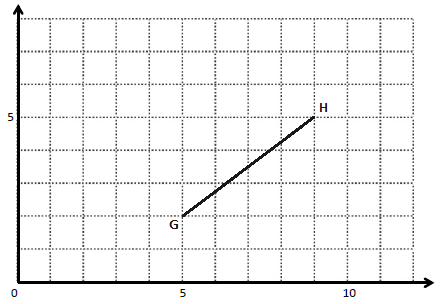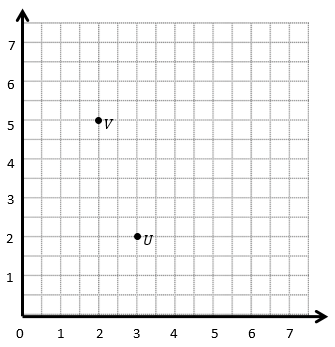# Patterns and the Coordinate Plane

## Objective

Draw perpendicular lines on a coordinate plane.

## Common Core Standards

### Core Standards

?

• 5.G.A.1 — Use a pair of perpendicular number lines, called axes, to define a coordinate system, with the intersection of the lines (the origin) arranged to coincide with the 0 on each line and a given point in the plane located by using an ordered pair of numbers, called its coordinates. Understand that the first number indicates how far to travel from the origin in the direction of one axis, and the second number indicates how far to travel in the direction of the second axis, with the convention that the names of the two axes and the coordinates correspond (e.g., x-axis and x-coordinate, y-axis and y-coordinate).

• 5.G.A.2 — Represent real world and mathematical problems by graphing points in the first quadrant of the coordinate plane, and interpret coordinate values of points in the context of the situation.

?

• 4.G.A.1

## Criteria for Success

?

1. Determine whether two line segments drawn on a grid or coordinate plane are perpendicular.
2. Draw perpendicular line segments on a grid or coordinate plane.
3. Identify the coordinates of points on two perpendicular line segments.
4. (Optional) Notice patterns in the coordinates of two perpendicular line segments.

## Tips for Teachers

?

Students have done similar work in Grade 4, in particularly Unit 4 Lesson 3, Lesson 15, and Lesson 17. This may still be a challenging lesson, though, so it may be useful to refer to Grade 4 Unit 4 to see the ways in which students worked on similar tasks to inform your instruction of this lesson.

#### Fishtank Plus

• Problem Set
• Student Handout Editor
• Vocabulary Package

?

### Problem 1

The Empire State Building is at the intersection of 5th Avenue and West 34th Street in New York City. Is the intersection of these streets perpendicular? Use the map below to help.#### References

Google Maps Map: Empire State Building

Modified by The Match Foundation, Inc.

### Problem 2

On the coordinate grid below, draw a line segment that is perpendicular to each given line segment.#### References

EngageNY Mathematics Grade 5 Mathematics > Module 6 > Topic C > Lesson 15Concept Development

Grade 5 Mathematics > Module 6 > Topic C > Lesson 15 of the New York State Common Core Mathematics Curriculum from EngageNY and Great Minds. © 2015 Great Minds. Licensed by EngageNY of the New York State Education Department under the CC BY-NC-SA 3.0 US license. Accessed Dec. 2, 2016, 5:15 p.m..

Modified by The Match Foundation, Inc.

### Problem 3

Use the coordinate plane below to answer the questions.1. What are the coordinates of points G and H?
2. Draw another line segment, ${\overline{JK}}$, on the coordinate plane that is also perpendicular and the same length.
3. What are the coordinates of point J and point K?
4. Explain how you figured out where to plot ${\overline{JK}}$.

#### References

EngageNY Mathematics Grade 5 Mathematics > Module 6 > Topic C > Lesson 16Concept Development

Grade 5 Mathematics > Module 6 > Topic C > Lesson 16 of the New York State Common Core Mathematics Curriculum from EngageNY and Great Minds. © 2015 Great Minds. Licensed by EngageNY of the New York State Education Department under the CC BY-NC-SA 3.0 US license. Accessed Dec. 2, 2016, 5:15 p.m..

Modified by The Match Foundation, Inc.

## Problem Set & Homework

#### Discussion of Problem Set

• In #1, how did you determine which sets of segments were perpendicular?
• In #2, do your segments look like your neighbor’s line segments? Are there other lines that are perpendicular to the given segments, or is your figure the only correct response?
• How is drawing perpendicular lines similar to and different from drawing parallel lines?
• In #3, are there other segments that are perpendicular to ${\overline{AB}}$? How do you know?

?

Use the coordinate plane below for #1 and #2.1. Draw a line through points and V.
2. Draw a second line that is perpendicular to $\overleftrightarrow{UV}$.

#### References

EngageNY Mathematics Grade 5 Mathematics > Module 6 > Topic C > Lesson 16Exit Ticket

Grade 5 Mathematics > Module 6 > Topic C > Lesson 16 of the New York State Common Core Mathematics Curriculum from EngageNY and Great Minds. © 2015 Great Minds. Licensed by EngageNY of the New York State Education Department under the CC BY-NC-SA 3.0 US license. Accessed Dec. 2, 2016, 5:15 p.m..

Modified by The Match Foundation, Inc.

?